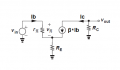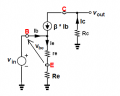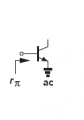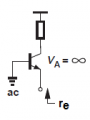# the voltage gain of a common emitter circuit

#### Ghina Bayyat

Joined Mar 11, 2018
135
i have a question about the voltage gain of a CE transistor
i know that Av = -Rc / Re
but in some websites i read that it's equal to Av = Beta * Rc /Rb
why is it different ? and what is the right one ? and why is there no - in the one i found ? is it just ignored or for a reason ?
i tried to figure it out by my self and i got :
Av = Vout / Vin
Av = Iout * Rout / Iin * Rin
as Iout = ic , Iin = ib and Rout = the resistor connected in series with the output then
Rout = Rc and Rin = the resistor connected in series with the input then Rin = RE
so we get Av = ic*rc / ib*re
Av = βib*rc / ib*re
then Av = β rc / re
and i got a new equation which is not exist anywhere
can u help me to know what is the right equation for Av and how to get it ?

Joined Mar 10, 2018
4,057

Last edited:

#### Bordodynov

Joined May 20, 2015
3,059

#### dl324

Joined Mar 30, 2015
15,536
can u help me to know what is the right equation for Av and how to get it ?
Circuits are usually designed so that beta variations won't affect the gain of the circuit and the gain is approximated as -Rc/Re. For sufficiently high values of beta, the error is small and masked by the tolerance of the resistors.

It would be helpful if you posted references to the other equation so we know the context in which it was given.

#### Ghina Bayyat

Joined Mar 11, 2018
135
but what i don't understand is how is it that Iin is ie ?? isn't it supposed to be ib ??
or is the equation Av = Iout *Rout / Iin *Rin WRONG ?? it can't be wrong

#### Ghina Bayyat

Joined Mar 11, 2018
135

#### Ghina Bayyat

Joined Mar 11, 2018
135
Circuits are usually designed so that beta variations won't affect the gain of the circuit and the gain is approximated as -Rc/Re. For sufficiently high values of beta, the error is small and masked by the tolerance of the resistors.
that's what i used to know but i wondered how did we get this ratio so i googled it but found different and complicated things and most websites said that Av = β Rc / Rb
so i tried to come up with the equation by the lows i have and got a more complicated equation
if as Bordodynov said and clearly it is right then where did ic , ie , and β go when we say
Av = Rc / Re ????

#### dl324

Joined Mar 30, 2015
15,536
that's what i used to know but i wondered how did we get this ratio so i googled it but found different and complicated things and most websites said that Av = β Rc / Rb
Cite some references so we can understand the context in which these statements were made.

It's not a good idea to design an amplifier to have it's gain dominated by beta. It's more common to use the minimum beta for a certain transistor type (e.g. 2N2222).

#### Jony130

Joined Feb 17, 2009
5,445
There are two popular small-signal BJT's model for CE amplifer.

The first one is a Hybrid-pi model

And for the CE amplifier with external RE resistor the small-signal model looks like this:Vout = - Ic*Rc = - Ib*β*Rc

Vin = Ib*r_pi + Ie *Re

Additional we know that Ie = (β + 1)*Ib

Therefore

Vin = Ib*r_pi + Ie *Re = Ib*r_pi + (β + 1)*Ib*Re = Ib*(r_pi + (β + 1)*Re)

So, the voltage gain is

Vout/Vin = - (β*Ib*Rc)/(Ib*(r_pi + (β + 1)*Re)) = - (βRc)/( r_pi + (β+1)*Re) (1) Ib cancel out.

But if we use an alternative small-signal model ( T-model ) the circuit will looks like this:And again

Vout = - Ic *Rc

And Vin = Ie*re + Ie*Re = Ie*(re + Re)

Aditional we know thet Ic = Ib*β and Ie = Ib + Ic = Ib + Ib*β = Ib*(β + 1) therefore Ic/Ie = (Ib*β)/(Ib*(β + 1)) = β/(β + 1).

From this we can write that Ic = Ie*β/(β + 1)

And the voltage gain is

Vout = - Ic *Rc = - Ie*β/(β + 1) *Rc
Vin = Ie*(re + Re)

Vout/Vin = -( Ie*β/(β + 1) *Rc)/ (Ie*(re + Re) = - Rc/(re + Re) *β/(β + 1) (2)

And because the beta is usually larger than 10 (10/11 = 0.909 ) we can ignore β/(β + 1) term in the gain formula and write Rc/(re + Re) where:

re = 26mV/Ie
r_pi = (β + 1)*re
Ie - emitter quiescent current
(based point current)

In some circuit re << RE so, we can skip re in the formula and we are left with Rc/Re
Also, we usually omit this "minus" sign because we know what this "minus" sign represents. This "minus" only informs us that the output voltage is the 180-degree phase shift with respect to the input voltage.

http://www.ittc.ku.edu/~jstiles/412/handouts/5.6 Small Signal Operation and Models/The Hybrid Pi and T Models lecture.pdf
http://www.ittc.ku.edu/~jstiles/412/handouts/5.6 Small Signal Operation and Models/section 5_6 Small Signal Operation and Models lecture.pdf

And those two voltage gain formulas will give you exactly the same result.

For the case without external RE resistor or if RE resistor was shorted by CE capacitor the gain formulas looks like this:

Av = - Rc/re *β/(β+1) ≈ - Rc/re

or

Av = - (β*Rc)/r_pi

Last edited:

#### Ghina Bayyat

Joined Mar 11, 2018
135
Cite some references so we can understand the context in which these statements were made.

It's not a good idea to design an amplifier to have it's gain dominated by beta. It's more common to use the minimum beta for a certain transistor type (e.g. 2N2222).
here are some sites :
https://www.elprocus.com/common-emitter-amplifier-circuit-working/
i know it's not a good idea to design a beta dependant transistor but i just wanna know how can we delete the part β / β +1 ? i mean it's a low and we can't remove parts of it like this the gain must be equal to -(Rc||RLoad)/(Re+re)*(β/(β+1) and if we delete the part β / β + 1 the value of the gain must be changed so how ?

#### Ghina Bayyat

Joined Mar 11, 2018
135
And because the beta is usually larger than 10 (10/11 = 0.909 ) we can ignore β/(β + 1) term in the gain formula and write Rc/(re + Re) where:
now i get it so because β / β+1 almost equals 1 then we ignore it
thank u so much i understand now
but the first circuit u put wasn't clear
any way i have one left question
it's kind of stupid but why do we say that ie is i in and re or Re is Rin ??
i always think isn't it supposed to be ib ??
and for what u said
re = 26mV/Ie
r_pi = (β + 1)*re
Ie - emitter quiescent current
(based point current)
aren't r-pi and re just different names for the same resistor ??

#### BobTPH

Joined Jun 5, 2013
6,527
What is β/(β+1) when β is large, say 300?

Bob

#### Jony130

Joined Feb 17, 2009
5,445
aren't r-pi and re just different names for the same resistor ??
No, not exactly the same. They are AC dynamic resistances. Which means that the re and r_pi does not represent any "real" resistance ( as ordinary resistor does) between the base and emitter.

In short. If you plot Ib=f(Vbe) and take a slope of this curve. The inverse of this given slope is a dynamic input resistance:

r_pi = hie = rbe = d(Vbe)/d(Ib)and we can say that the r_pi is an input resistance looking into the base with emitter terminal at AC ground

r_pi = d(Vbe)/d(Ib) = Vt/Ib = (β + 1)*re remember that re is Vt/Ie and Ie = (β + 1)*Ib

On the other hand re is an input resistance looking into the emitter terminal with the base terminal at AC ground.re = d(Vbe)/d(Ie) = Vt/Ie = 26mV/Ie

it's kind of stupid but why do we say that ie is i in and re or Re is Rin ??
i always think isn't it supposed to be ib ??
In T-model we are looking at the circuit form the emitter current point of view. But Ib is still an input current.

But we can transform it onto the emitter node vbe/Ie = re or Ie = vbe/re ; Ib = Ie - Ic

Also, kept in mind that all of this is just a small-signal AC model. And the key word here is "model" a fictional representation of a circuit.

Last edited:

#### BobaMosfet

Joined Jul 1, 2009
2,073
Just a really simple over-view for the original poster-

I think it's important to remember some basic things about BJTs. BJTs are not conventional current-flow devices. They are labeled specifically as they are because of the real direction that current flows (from negative to positive). The emitter emits INTO the junction, towards the collector. The collect catches what the emitter sends. The base controls the magnitude of that transmission.

Ie = Emitter current
Ib = Base current
Ic = Collector current.

BJTs are current-controlled devices. FETs are potential (aka voltage)-controlled devices. (We don't discus FETs here).

Your alpha gain, represents the gain across your junction (from emitter to collector).
Wherever the term 'gain' is used, we really mean 'difference'.

Your emitter, as it supplies all current through the BJT, will always be a summation of the current at the collector, and the base.

Ie = Ib + Ic

Because Alpha is merely a value describing the difference in current levels between the collect and the emitter, it will be close to 1, but can never actually be 1, because some of the current leaving the emitter, goes to the base.

This leaves us with Beta (also called hFE on datasheets):

B = Ic / Ib

The idea here, is that an incredibly minuscule current on the base, will cause an enormous current flow between the emitter and the collector. In fact, it's hard in the beginning for people learning about transistors to fully grasp the scale of difference between the base and the collector. Transistors are extremely sensitive. As such, a few uA (millionths of an amp) could cause several mA or 10's or 100's of mA (thousanths of an Amp) change at the collector. The main reason we want Beta stable, is so that in any given situation, that ratio between the collector and the base will stay fairly consistent-- so we can count on it always magnifying our base signal to an expected value (or close).

Hopefully, by understanding the basic relationships between B, C, and E, and the underlying rudimentary formulaic relationships for gain and why, you will be able to better understand the other arithmetic and formulas others have shared above.

So when you see Av = Beta * Rc/Rb, what you're really seeing is Av = Ic/Ib * Rc/Rb -- What is Ohm's law? E = I*R. Do you see Ohm's Law in the formula you are asking a question about? You should.

Joined Mar 10, 2018
4,057
Actually BJTs are V controlled devices, defining equation -The collector current is a function of Vbe. But then we have the approximation
of Icollector = beta X Ibase.

The device can be used and thought of either way.

The ultimate wrench in these arguments is you cannot have current without voltage,
but you can have voltage w/o current, a simple field. So voltage is the independent
variable in this argument.

A more in depth thread on the topic (get out the aspirin) -

https://www.eevblog.com/forum/beginners/current-controlled-device-vs-voltage-controlled/

Regards, Dana.

#### Ghina Bayyat

Joined Mar 11, 2018
135
What is β/(β+1) when β is large, say 300?

Bob
yes i got it because it's close to 1 thank u

#### Ghina Bayyat

Joined Mar 11, 2018
135
No, not exactly the same. They are AC dynamic resistances. Which means that the re and r_pi does not represent any "real" resistance ( as ordinary resistor does) between the base and emitter.

In short. If you plot Ib=f(Vbe) and take a slope of this curve. The inverse of this given slope is a dynamic input resistance:

r_pi = hie = rbe = d(Vbe)/d(Ib)and we can say that the r_pi is an input resistance looking into the base with emitter terminal at AC ground

r_pi = d(Vbe)/d(Ib) = Vt/Ib = (β + 1)*re remember that re is Vt/Ie and Ie = (β + 1)*Ib

On the other hand re is an input resistance looking into the emitter terminal with the base terminal at AC ground.re = d(Vbe)/d(Ie) = Vt/Ie = 26mV/Ie
i understand and also re is constant and doesn't change with frequency right ?
In T-model we are looking at the circuit form the emitter current point of view. But Ib is still an input current.

But we can transform it onto the emitter node vbe/Ie = re or Ie = vbe/re ; Ib = Ie - Ic

Also, kept in mind that all of this is just a small-signal AC model. And the key word here is "model" a fictional representation of a circuit.
what u said i got all but what how can we transform ib onto the emitter node ? i mean u said ib is still the input current but since we're looking at the circuit from the emitter current point of view so we can transform ib onto the emitter node but what do we actually do ? is it just normal that we change the actual input current ?

#### Ghina Bayyat

Joined Mar 11, 2018
135
Just a really simple over-view for the original poster-

I think it's important to remember some basic things about BJTs. BJTs are not conventional current-flow devices. They are labeled specifically as they are because of the real direction that current flows (from negative to positive). The emitter emits INTO the junction, towards the collector. The collect catches what the emitter sends. The base controls the magnitude of that transmission.

Ie = Emitter current
Ib = Base current
Ic = Collector current.

BJTs are current-controlled devices. FETs are potential (aka voltage)-controlled devices. (We don't discus FETs here).

Your alpha gain, represents the gain across your junction (from emitter to collector).
Wherever the term 'gain' is used, we really mean 'difference'.

Your emitter, as it supplies all current through the BJT, will always be a summation of the current at the collector, and the base.

Ie = Ib + Ic

Because Alpha is merely a value describing the difference in current levels between the collect and the emitter, it will be close to 1, but can never actually be 1, because some of the current leaving the emitter, goes to the base.

This leaves us with Beta (also called hFE on datasheets):

B = Ic / Ib

The idea here, is that an incredibly minuscule current on the base, will cause an enormous current flow between the emitter and the collector. In fact, it's hard in the beginning for people learning about transistors to fully grasp the scale of difference between the base and the collector. Transistors are extremely sensitive. As such, a few uA (millionths of an amp) could cause several mA or 10's or 100's of mA (thousanths of an Amp) change at the collector. The main reason we want Beta stable, is so that in any given situation, that ratio between the collector and the base will stay fairly consistent-- so we can count on it always magnifying our base signal to an expected value (or close).

Hopefully, by understanding the basic relationships between B, C, and E, and the underlying rudimentary formulaic relationships for gain and why, you will be able to better understand the other arithmetic and formulas others have shared above.

So when you see Av = Beta * Rc/Rb, what you're really seeing is Av = Ic/Ib * Rc/Rb -- What is Ohm's law? E = I*R. Do you see Ohm's Law in the formula you are asking a question about? You should.
thanks good explanation and i understand this equation but my problem is why is it that some times they say Av = β Rc / Rb and some times it is Rc / Re
i was thinking that ib and rb are the input resistor and current so why is it rc / re
i understand now that it is because we're looking from the emitter point of view but how do we determine when to look at the circuit from the emitter or the base point of view ? because of this now we have two equations for the voltage gain Av = β rc / rb and Av = rc / re and of course their results are different

#### Ghina Bayyat

Joined Mar 11, 2018
135
Actually BJTs are V controlled devices, defining equation -The collector current is a function of Vbe. But then we have the approximation
of Icollector = beta X Ibase.

The device can be used and thought of either way.

The ultimate wrench in these arguments is you cannot have current without voltage,
but you can have voltage w/o current, a simple field. So voltage is the independent
variable in this argument.

A more in depth thread on the topic (get out the aspirin) -

https://www.eevblog.com/forum/beginners/current-controlled-device-vs-voltage-controlled/

Regards, Dana.
WHAT IS ALL OF THIS ???????????
i'm lost now i only asked a simple question and i found vvveerrryy big answers
we're not discussing voltage controlled or current controlled devices
i just wanna know how could it be that the circuit has two equations for Av and the two of them have different results of course but the two of them are right ?

#### Jony130

Joined Feb 17, 2009
5,445
what u said i got all but what how can we transform ib onto the emitter node ? i mean u said ib is still the input current but since we're looking at the circuit from the emitter current point of view so we can transform ib onto the emitter node but what do we actually do ? is it just normal that we change the actual input current ?
(β+1) is a "transformation factor", any resistance at the base is seen (reflect it to the emitter) at the emitter as a β + 1 smaller resistance ( re = r_pi/(β + 1 ) ).
And any resistance at the emitter is seen at the base node as a (β + 1) large resistance r_pi = ( β + 1)*re

also re is constant and doesn't change with frequency right ?
Yes, but BJT also have Cbe capacitance. So at hight frequency we have a complex impedance.